‘MCMCvis’ package

Intro

MCMCvis is an R package used to visualize, manipulate, and summarize MCMC output. This may be Bayesian model output fit with Stan, NIMBLE, JAGS, and other software.

The package contains six functions:

• MCMCsummary - summarize MCMC output for particular parameters of interest
• MCMCpstr - summarize MCMC output and extract posterior chains for particular parameters of interest while preserving parameter structure
• MCMCtrace - create trace and density plots of MCMC chains for particular parameters of interest
• MCMCchains - extract posterior chains from MCMC output for particular parameters of interest
• MCMCplot - create caterpillar plots from MCMC output for particular parameters of interest
• MCMCdiag - create a .txt file and save specified objects that summarize model inputs, outputs, and diagnostics

MCMCvis was designed to perform key functions for MCMC analysis using minimal code, in order to free up time/brainpower for interpretation of analysis results. Functions support simple and straightforward subsetting of model parameters within the calls, and produce presentable, ‘publication-ready’ output.

All functions in the package accept stanfit objects (created with the rstan package), stanreg objects (created with the rstanarm package), brmsfit objects (created with the brms package), mcmc.list objects (created with the rjags or coda packages), mcmc objects (created with the coda or nimble packages), list objects (created with the nimble package), R2jags output (created with the R2jags package), jagsUI output (created with the jagsUI package), and matrices of MCMC output (chains combined into a single column per parameter - columns to be named with parameter names). The functions automatically detect the object type and proceed accordingly. Model output objects can be inserted directly into the MCMCvis functions as an argument.

JAGS model

library(rjags)

# create JAGS model
mf <- "
model {
for (i in 1:10)
{
y[i] ~ dnorm(mu, 0.01);
}
mu ~ dnorm(0, 0.01)
}
"

data <- list(y = rnorm(10))

jm <- rjags::jags.model(
textConnection(mf),
data = data,
n.chains = 3)

jags_out <- rjags::coda.samples(
jm,
variable.names = 'mu',
n.iter = 500)
library(MCMCvis)
MCMCsummary(jags_out, round = 2)
##     mean   sd  2.5%   50% 97.5% Rhat n.eff
## mu -0.28 2.97 -6.13 -0.14  5.22    1  1397

NIMBLE model

library(nimble)

# create NIMBLE model
mf2 <- nimbleCode({
for (i in 1:10) {
y[i] ~ dnorm(mu, 0.01);
}
mu ~ dnorm(0, 0.01)
})

nimble_out <- nimbleMCMC(
code = mf2,
constants = list(N = 10),
data = data,
inits = list(mu = 0),
nchains = 4,
niter = 500)
MCMCsummary(nimble_out, round = 2)
##     mean   sd  2.5%   50% 97.5% Rhat n.eff
## mu -0.03 2.99 -5.76 -0.1  5.88    1  2000

Stan model

library(rstan)

# create Stan model
sm <- "
data {
real y;
}
parameters {
real mu;
}
model {
for (i in 1:10) {
y[i] ~ normal(mu, 10);
}
mu ~ normal(0, 10);
}
"

stan_out <- stan(
model_code = sm,
data = data,
iter = 500)
MCMCsummary(stan_out, round = 2)
##       mean   sd  2.5%   50% 97.5% Rhat n.eff
## mu   -0.51 2.82 -6.06 -0.36  5.07 1.01   414
## lp__ -0.45 0.61 -2.27 -0.23 -0.01 1.00   508

MCMCsummary

MCMCsummary is used to output summary information from MCMC output as a data.frame. All digits are reported by default.

We’ll use the built in mcmc.list object for the examples below, but model output of any of the supported types will behave in the same way.

MCMCsummary(MCMC_data)
##                mean        sd       2.5%        50%      97.5% Rhat n.eff
## alpha  -9.775030  2.087347 -13.873736  -9.747235  -5.718132    1 10406
## alpha -10.954077  4.087058 -18.986880 -10.996852  -2.932785    1 10807
## alpha -12.812863  5.345266 -23.334561 -12.833156  -2.505842    1 10500
## alpha -13.162363  4.549087 -22.002841 -13.131785  -4.270300    1 11160
## alpha -11.683834  8.888936 -28.857696 -11.734387   6.059936    1 10253
## alpha  -8.272228  6.200246 -20.263314  -8.218744   3.889713    1 10500
## beta   -4.618601  6.544247 -17.193329  -4.619954   8.378438    1 10411
## beta  -14.169569  6.633089 -27.152037 -14.084978  -1.414025    1 10500
## beta  -35.936571  8.415110 -52.599736 -35.998599 -19.259210    1 10884
## beta    6.172961 10.716345 -14.670444   6.110057  27.266086    1 10500
## beta    8.423471  3.463758   1.625696   8.447591  15.125755    1 10500
## beta  -12.051330  2.336788 -16.655170 -12.046641  -7.537826    1 10500

The number of decimal places displayed can be specified with round (except for Rhat which is always rounded to 2 decimal places and n.eff which always displays whole numbers). Alternatively, the significant digits displayed can be specified with digits.

MCMCsummary(MCMC_data, round = 2)
##            mean    sd   2.5%    50%  97.5% Rhat n.eff
## alpha  -9.78  2.09 -13.87  -9.75  -5.72    1 10406
## alpha -10.95  4.09 -18.99 -11.00  -2.93    1 10807
## alpha -12.81  5.35 -23.33 -12.83  -2.51    1 10500
## alpha -13.16  4.55 -22.00 -13.13  -4.27    1 11160
## alpha -11.68  8.89 -28.86 -11.73   6.06    1 10253
## alpha  -8.27  6.20 -20.26  -8.22   3.89    1 10500
## beta   -4.62  6.54 -17.19  -4.62   8.38    1 10411
## beta  -14.17  6.63 -27.15 -14.08  -1.41    1 10500
## beta  -35.94  8.42 -52.60 -36.00 -19.26    1 10884
## beta    6.17 10.72 -14.67   6.11  27.27    1 10500
## beta    8.42  3.46   1.63   8.45  15.13    1 10500
## beta  -12.05  2.34 -16.66 -12.05  -7.54    1 10500

Specific parameters can be specified to subset summary information. Square brackets in parameter names are ignored by default. For instance, all alpha parameters can be plotted using params = 'alpha'.

MCMCsummary(MCMC_data,
params = 'alpha',
round = 2)
##            mean   sd   2.5%    50% 97.5% Rhat n.eff
## alpha  -9.78 2.09 -13.87  -9.75 -5.72    1 10406
## alpha -10.95 4.09 -18.99 -11.00 -2.93    1 10807
## alpha -12.81 5.35 -23.33 -12.83 -2.51    1 10500
## alpha -13.16 4.55 -22.00 -13.13 -4.27    1 11160
## alpha -11.68 8.89 -28.86 -11.73  6.06    1 10253
## alpha  -8.27 6.20 -20.26  -8.22  3.89    1 10500

Individual parameters can also be specified. For example, one alpha (of many) may be specified. In this case, the square brackets should not be ignored, so that only the alpha parameter can be specified. Use the argument ISB = FALSE to prevent square brackets from being ignored (ISB is short for ‘Ignore Square Brackets’). The exact argument can be used to specify whether the parameter name should be matched exactly (after taking into account the ISB argument). Therefore, to return a single alpha parameter, ISB = FALSE and exact = TRUE can be used in the following way.

MCMCsummary(MCMC_data,
params = 'alpha',
ISB = FALSE,
exact = TRUE,
round = 2)
##           mean   sd   2.5%   50% 97.5% Rhat n.eff
## alpha -9.78 2.09 -13.87 -9.75 -5.72    1 10406

When exact = FALSE, the params argument reads like a regular expression. Because of this, square brackets must be escaped with \\. All other regular expression syntax is accepted, as typically applied in R. This can be useful for returning a specific set of a large number of parameters. A great tools for regular expressions in R can be found here (though note two slashes are needed to escape characters in this function rather than one, hence \\[ as opposed to \[). \\d can be used to specify any digits in a particular place.

MCMCsummary(MCMC_data,
params = 'alpha\\[1\\]',
ISB = FALSE,
exact = FALSE,
round = 2)
##           mean   sd   2.5%   50% 97.5% Rhat n.eff
## alpha -9.78 2.09 -13.87 -9.75 -5.72    1 10406

When using exact = FALSE, if alpha has 10 or more parameters (i.e., more than two digits for the index), the | (OR) may be needed. For instance, while one could use alpha[1:10] to select the first ten indices of the vector alpha in R, the regex equivalent to do the same with MCMCvis would be alpha\\[(\\d|)\\]. The \\d specifies any digit, the | represents OR (so any one digit number OR), the  specifies that the first digit must be one, and the  specifies that the second digit must be zero (so return any one digit number, or 10, resulting in the equivalent of alpha[1:10]). Ranges for each digit can also be specified. For instance, the regex equivalent of the R alpha[5:15] would be alpha\\[([5-9]|[0-5])\\].

The excl argument can be used to exclude any parameters. For instance, if all parameters are desired except for the alphas, use params = 'all', excl = 'alpha', ISB = FALSE, exact = FALSE. These arguments can be used in any of the functions in the package.

MCMCsummary(MCMC_data,
params = 'all',
excl = 'alpha',
ISB = FALSE,
exact = FALSE,
round = 2)
##           mean    sd   2.5%    50%  97.5% Rhat n.eff
## beta  -4.62  6.54 -17.19  -4.62   8.38    1 10411
## beta -14.17  6.63 -27.15 -14.08  -1.41    1 10500
## beta -35.94  8.42 -52.60 -36.00 -19.26    1 10884
## beta   6.17 10.72 -14.67   6.11  27.27    1 10500
## beta   8.42  3.46   1.63   8.45  15.13    1 10500
## beta -12.05  2.34 -16.66 -12.05  -7.54    1 10500

Setting the Rhat and n.eff arguments to FALSE can be used to avoid calculating the Rhat statistic and number of effective samples, respectively (defaults for both Rhat and n.eff are TRUE). Specifying FALSE may greatly increase function speed with very large mcmc.list objects. Values for Rhat near 1 suggest convergence (Brooks and Gelman 1998). Rhat and n.eff values for mcmc.list objects are calculated using the coda package (what is typically returned by packages that utilize JAGS). Rhat and n.eff values for stanfit and jagsUI objects are calculated using a ‘split chain’ Rhat (as used by their respective packages). The approaches differ slightly between the coda and stanfit/jagsUI packages. Details on calculation of Rhat and number of effective samples using rstan can be found in the Stan manual (Stan Development Team 2018).

MCMCsummary(MCMC_data,
params = 'alpha',
Rhat = TRUE,
n.eff = TRUE,
round = 2)
##            mean   sd   2.5%    50% 97.5% Rhat n.eff
## alpha  -9.78 2.09 -13.87  -9.75 -5.72    1 10406
## alpha -10.95 4.09 -18.99 -11.00 -2.93    1 10807
## alpha -12.81 5.35 -23.33 -12.83 -2.51    1 10500
## alpha -13.16 4.55 -22.00 -13.13 -4.27    1 11160
## alpha -11.68 8.89 -28.86 -11.73  6.06    1 10253
## alpha  -8.27 6.20 -20.26  -8.22  3.89    1 10500

Sample quantiles in MCMCsummary can now be specified directly using the probs argument, removing the need to define custom quantiles with the func argument. The default behavior is to provide 2.5%, 50%, and 97.5% quantiles. These probabilities can be changed by supplying a numeric vector to the probs argument.

MCMCsummary(MCMC_data,
params = 'alpha',
Rhat = TRUE,
n.eff = TRUE,
probs = c(0.1, 0.5, 0.9),
round = 2)
##            mean   sd    10%    50%   90% Rhat n.eff
## alpha  -9.78 2.09 -12.47  -9.75 -7.13    1 10406
## alpha -10.95 4.09 -16.18 -11.00 -5.67    1 10807
## alpha -12.81 5.35 -19.76 -12.83 -6.10    1 10500
## alpha -13.16 4.55 -19.06 -13.13 -7.29    1 11160
## alpha -11.68 8.89 -23.03 -11.73 -0.36    1 10253
## alpha  -8.27 6.20 -16.34  -8.22 -0.31    1 10500

Setting HPD = TRUE will cause MCMCsummary to use HPDinterval from the coda package to compute highest posterior density intervals based on the probability specified in the hpd_prob argument (this argument is different than probs argument, which is reserved for quantiles). Note that for each parameter HPDinterval normally returns one interval per chain. However, MCMCsummary first pools the chains, forcing HPDinterval to compute a single interval across all posterior samples for each parameter. This step is done for user convenience.

MCMCsummary(MCMC_data,
params = 'alpha',
Rhat = TRUE,
n.eff = TRUE,
HPD = TRUE,
hpd_prob = 0.8,
round = 2)
##            mean   sd 80%_HPDL 80%_HPDU Rhat n.eff
## alpha  -9.78 2.09   -12.38    -7.05    1 10406
## alpha -10.95 4.09   -16.03    -5.58    1 10807
## alpha -12.81 5.35   -19.80    -6.15    1 10500
## alpha -13.16 4.55   -19.44    -7.74    1 11160
## alpha -11.68 8.89   -23.18    -0.57    1 10253
## alpha  -8.27 6.20   -16.14    -0.15    1 10500

The func argument can be used to return metrics of interest not already returned by default for MCMCsummary. Input is a function to be performed on posteriors for each specified parameter. Values returned by the function will be displayed as a column in the summary output (or multiple columns if the function returns more than one value). In this way, functions from other packages can be used to derive metrics of interest on posterior output. Column name(s) for function output can be specified with the func_name argument. The example below uses the empirical cumulative distribution function ecdf to compute the proportion of posterior samples that are less than -10 for each alpha parameter. The argument pg0 can be used to specify whether to calculate the proportion of the posterior that is greater than 0.

MCMCsummary(MCMC_data,
params = 'alpha',
Rhat = TRUE,
n.eff = TRUE,
round = 2,
func = function(x) ecdf(x)(-10), func_name = "ecdf-10",
pg0 = TRUE)
##            mean   sd   2.5%    50% 97.5% Rhat n.eff  p>0 ecdf-10
## alpha  -9.78 2.09 -13.87  -9.75 -5.72    1 10406 0.00    0.45
## alpha -10.95 4.09 -18.99 -11.00 -2.93    1 10807 0.00    0.60
## alpha -12.81 5.35 -23.33 -12.83 -2.51    1 10500 0.01    0.70
## alpha -13.16 4.55 -22.00 -13.13 -4.27    1 11160 0.00    0.75
## alpha -11.68 8.89 -28.86 -11.73  6.06    1 10253 0.09    0.58
## alpha  -8.27 6.20 -20.26  -8.22  3.89    1 10500 0.09    0.39

MCMCpstr

MCMCpstr is used to output summary information and posterior chains from MCMC output while preserving the original structure of the specified parameters (i.e., scalar, vector, matrix, array). Preserving the original structure can be helpful when plotting or summarizing parameters with multidimensional structure. Particular parameters of interest can be specified as with other functions with the params argument.

Function output has two types. When type = 'summary' (the default), a list with calculated values for each specified parameter is returned, similar to output obtained when fitting models with the jags.samples function (as opposed to coda.samples) from the rjags package.

The function calculates summary information only for the specified function. The function to be used is specified using the func argument.

MCMCpstr(MCMC_data,
params = 'alpha',
func = mean,
type = 'summary')
## \$alpha
##   -9.775030 -10.954077 -12.812863 -13.162363 -11.683834  -8.272228

Custom functions can be specified as well. If the output length of the specified function is greater than 1 when type = 'summary', an extra dimension is added to the function output. For instance, a vector becomes a matrix, a matrix a three dimensional array, and so forth.

MCMCpstr(MCMC_data, func = function(x) quantile(x, probs = c(0.01, 0.99)))
## \$alpha
##                 1%        99%
## alpha -14.74923 -5.0484174
## alpha -20.43502 -1.5155018
## alpha -25.32232 -0.4924355
## alpha -23.58314 -2.4859330
## alpha -32.38629  8.8685816
## alpha -22.56589  6.0892554
##
## \$beta
##                1%        99%
## beta -19.75530  10.840028
## beta -30.05090   1.018119
## beta -55.61435 -16.135057
## beta -18.86956  30.939547
## beta   0.48582  16.198588
## beta -17.60307  -6.658913

When type = 'chains', a list with posterior chain values for each specified parameter is returned. The structure of the parameter is preserved - posterior chain values are concatenated and placed in an additional dimension. For instance, output for a vector parameter will be in matrix format for that element of the list. Similarly, output for a matrix parameter will be in a three dimensional array.

ex <- MCMCpstr(MCMC_data, type = 'chains')
dim(ex\$alpha)
##      6 10500

MCMCtrace

MCMCtrace is used to create trace and density plots for MCMC output. This is useful for diagnostic purposes. Particular parameters can also be specified, as with MCMCsummary. Output is written to PDF by default to enable more efficient review of posteriors - this also reduces computation time. PDF output is particularly recommended for large numbers of parameters. pdf = FALSE can be used to prevent output to PDF.

MCMCtrace(MCMC_data,
params = c('beta', 'beta', 'beta'),
ISB = FALSE,
exact = TRUE,
pdf = FALSE)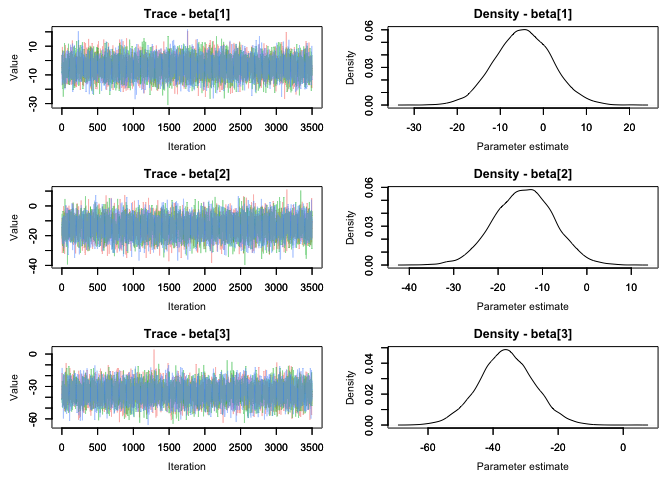Just trace plots can be plotted with type = 'trace'. Just density plots can be plotted with type = 'density'. Default is type = 'both' which outputs both trace and density plots. Density plots for individual chains can be output using the ind argument.

MCMCtrace(MCMC_data,
params = 'beta',
type = 'density',
ind = TRUE,
pdf = FALSE)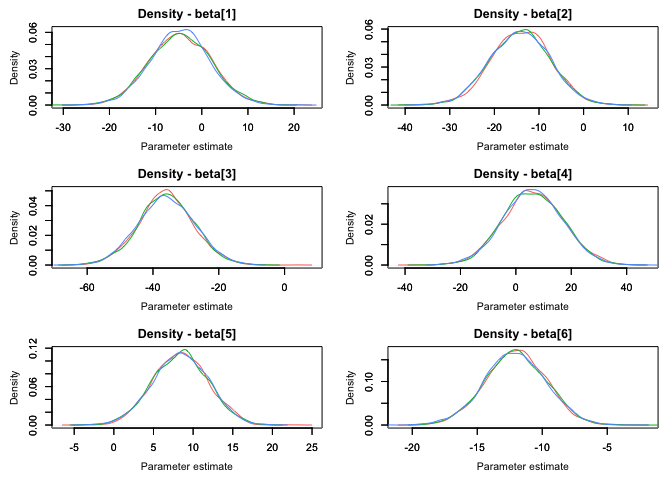The PDF document will be output to the current working directory by default, but another directory can be specified. The open_pdf argument can be used to prevent the produced PDF from opening in a viewer once generated.

MCMCtrace(MCMC_data,
pdf = TRUE,
open_pdf = FALSE,
filename = 'MYpdf',
wd = 'DIRECTORY_HERE')

iter denotes how many iterations should be plotted for the chain the trace and density plots. The default is 5000, meaning that the last 5000 iterations of each chain are plotted. Remember, this is the final posterior chain, not including the specified burn-in or warm-up (specified when the model was run). If less than 5000 iterations are run, the full number of iterations will be plotted.

MCMCtrace(MCMC_data,
params = c('beta', 'beta', 'beta'),
ISB = FALSE,
exact = TRUE,
iter = 100,
ind = TRUE,
pdf = FALSE)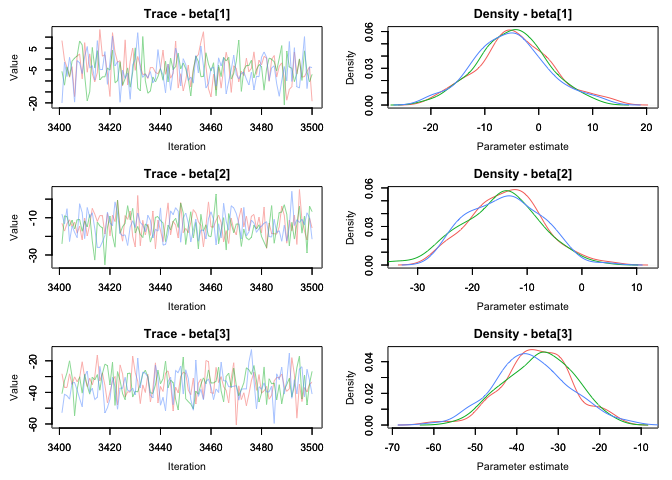Overlap between the priors and posteriors (PPO - prior posterior overlap) can also be calculated by specifying the priors associated with each parameter using the priors argument. This is particularly useful when investigating how large the effect of the prior is on the posterior distribution - this can be informative when trying to determine how identifiable a particular parameter is in a model.

The priors argument takes a matrix as input, with each column representing a prior for a different parameter and each row representing a random draw from that prior distribution. These draws can be generated using R functions such as rnorm, rgamma, runif, etc. Parameters are plotted alphabetically - priors should be sorted accordingly. If the priors argument contains only one prior and more than one parameter is specified for the params argument, this prior will be used for all parameters. The number of draws for each prior should equal the number of iterations specified by (or total draws if less than ) times the number of chains, though the function will automatically adjust if more or fewer iterations are specified. It is important to note that some discrepancies between MCMC samplers and R may exist regarding the parameterization of distributions - one example of this is the use of precision in JAGS but standard deviation in R and Stan for the ‘second parameter’ of the normal distribution. Values for Rhat and number of effective samples can be plotting on the density plots using the Rhat and n.eff arguments.

# note that the same prior used for all parameters
# the following prior is equivalent to dnorm(0, 0.001) in JAGS
PR <- rnorm(15000, 0, 32)

MCMCtrace(MCMC_data,
params = c('beta', 'beta', 'beta'),
ISB = FALSE,
exact = TRUE,
priors = PR,
pdf = FALSE,
Rhat = TRUE,
n.eff = TRUE)
## Warning in MCMCtrace(MCMC_data, params = c("beta", "beta", "beta"), :
## Only one prior specified for > 1 parameter. Using a single prior for all
## parameters.
## Warning in MCMCtrace(MCMC_data, params = c("beta", "beta", "beta"), :
## Number of samples in prior is greater than number of total or specified
## iterations (for all chains) for specified parameter. Only last 10500 iterations
## will be used.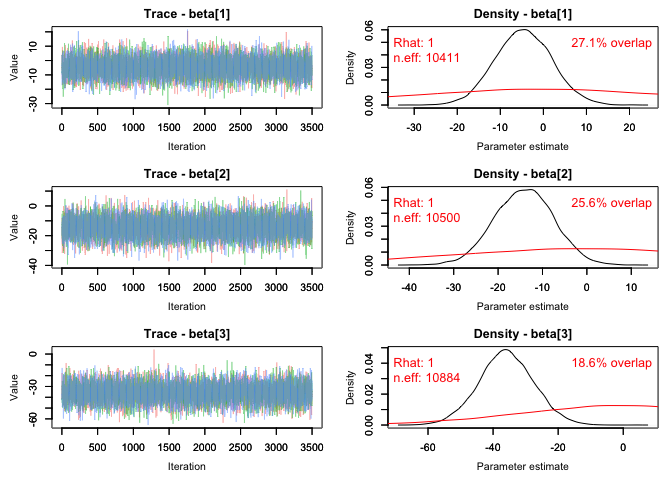Plots can be scaled to visualize both the posterior and the prior distribution using the post_zm argument.

MCMCtrace(MCMC_data,
params = c('beta', 'beta', 'beta'),
ISB = FALSE,
exact = TRUE,
priors = PR,
pdf = FALSE,
post_zm = FALSE)
## Warning in MCMCtrace(MCMC_data, params = c("beta", "beta", "beta"), :
## Only one prior specified for > 1 parameter. Using a single prior for all
## parameters.
## Warning in MCMCtrace(MCMC_data, params = c("beta", "beta", "beta"), :
## Number of samples in prior is greater than number of total or specified
## iterations (for all chains) for specified parameter. Only last 10500 iterations
## will be used.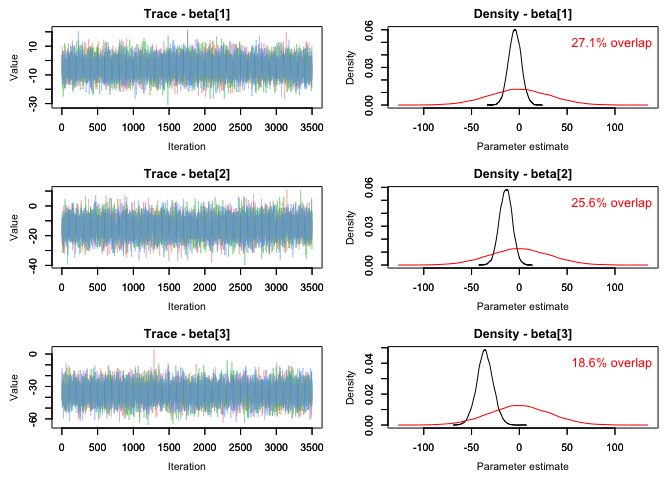Percent overlap can be output to an R object as well using the PPO_out argument. Plotting of the trace plots can be suppressed with plot = FALSE.

PPO <- MCMCtrace(MCMC_data,
params = c('beta', 'beta', 'beta'),
ISB = FALSE,
exact = TRUE,
priors = PR,
plot = FALSE,
PPO_out = TRUE)
## Warning in MCMCtrace(MCMC_data, params = c("beta", "beta", "beta"), :
## Only one prior specified for > 1 parameter. Using a single prior for all
## parameters.
## Warning in MCMCtrace(MCMC_data, params = c("beta", "beta", "beta"), :
## Number of samples in prior is greater than number of total or specified
## iterations (for all chains) for specified parameter. Only last 10500 iterations
## will be used.
PPO
##     param percent_PPO
## 1 beta        27.1
## 2 beta        25.6
## 3 beta        18.6

Additional arguments can be used to change the limits of the density plots, axes labels, plot titles, line width and type, size and color of text, tick and axes label size, position of ticks, color of lines, and thickness of axes.

MCMCtrace(MCMC_data,
params = c('beta', 'beta', 'beta'),
ISB = FALSE,
exact = TRUE,
priors = PR,
pdf = FALSE,
Rhat = TRUE,
n.eff = TRUE,
xlab_tr = 'This is the x for trace',
ylab_tr = 'This is the y for trace',
main_den = 'Custom density title',
lwd_den = 3,
lty_pr = 2,
col_pr = 'green',
sz_txt = 1.3,
sz_ax = 2,
sz_ax_txt = 1.2,
sz_tick_txt = 1.2,
sz_main_txt = 1.3)
## Warning in MCMCtrace(MCMC_data, params = c("beta", "beta", "beta"), :
## Only one prior specified for > 1 parameter. Using a single prior for all
## parameters.
## Warning in MCMCtrace(MCMC_data, params = c("beta", "beta", "beta"), :
## Number of samples in prior is greater than number of total or specified
## iterations (for all chains) for specified parameter. Only last 10500 iterations
## will be used.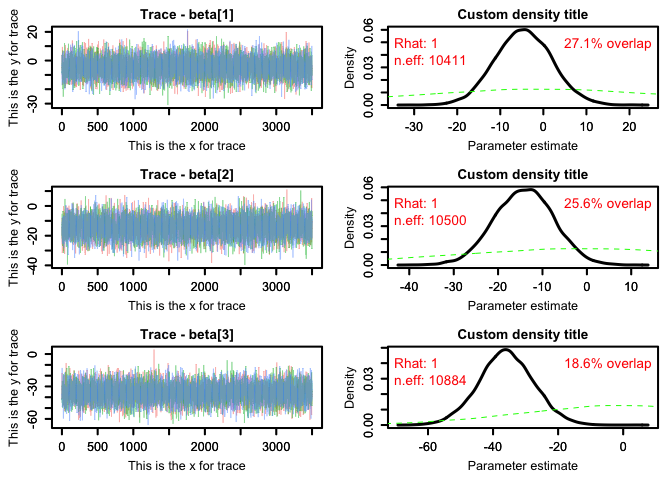If simulated data were used to fit the model, the generating values used to simulate the data can be specified using the gvals argument. This makes it possible to compare posterior estimates with the true parameter values. Generating values will be displayed as vertical dotted lines. Similar to the priors argument, if one value is specified when more than one parameter is used, this one generating value will be used for all parameters.

# generating values for each parameter used to simulate data
GV <- c(-10, -5.5, -15)

MCMCtrace(MCMC_data,
params = c('beta', 'beta', 'beta'),
ISB = FALSE,
exact = TRUE,
gvals = GV,
pdf = FALSE)